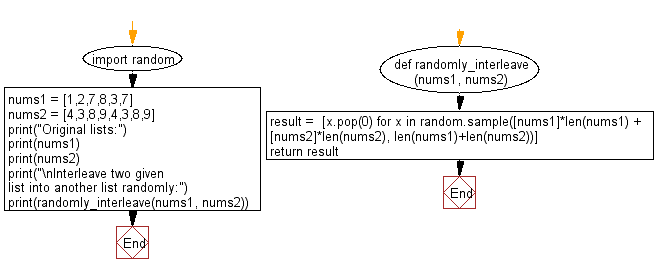﻿ Python: Interleave two given list into another list randomly - w3resource# Python: Interleave two given list into another list randomly

## Python List: Exercise - 147 with Solution

Write a Python program to interleave two given list into another list randomly.

Sample Solution:

Python Code:

``````import random
def randomly_interleave(nums1, nums2):
result =  [x.pop(0) for x in random.sample([nums1]*len(nums1) + [nums2]*len(nums2), len(nums1)+len(nums2))]
return result
nums1 = [1,2,7,8,3,7]
nums2 = [4,3,8,9,4,3,8,9]
print("Original lists:")
print(nums1)
print(nums2)
print("\nInterleave two given list into another list randomly:")
print(randomly_interleave(nums1, nums2))
```
```

Sample Output:

```Original lists:
[1, 2, 7, 8, 3, 7]
[4, 3, 8, 9, 4, 3, 8, 9]

Interleave two given list into another list randomly:
[4, 1, 2, 3, 8, 9, 4, 3, 7, 8, 9, 8, 3, 7]
```

Flowchart:## Visualize Python code execution:

The following tool visualize what the computer is doing step-by-step as it executes the said program:

Python Code Editor:

Have another way to solve this solution? Contribute your code (and comments) through Disqus.

What is the difficulty level of this exercise?

Test your Python skills with w3resource's quiz

﻿

## Python: Tips of the Day

```print(2_000_000)
```2000000